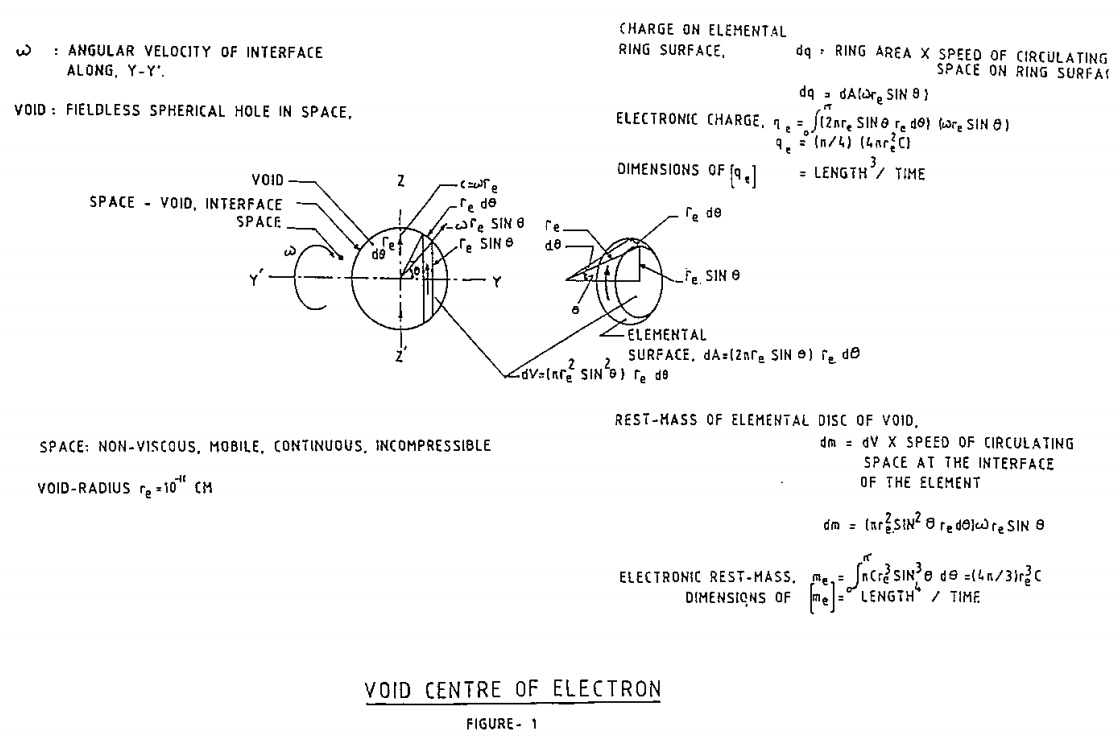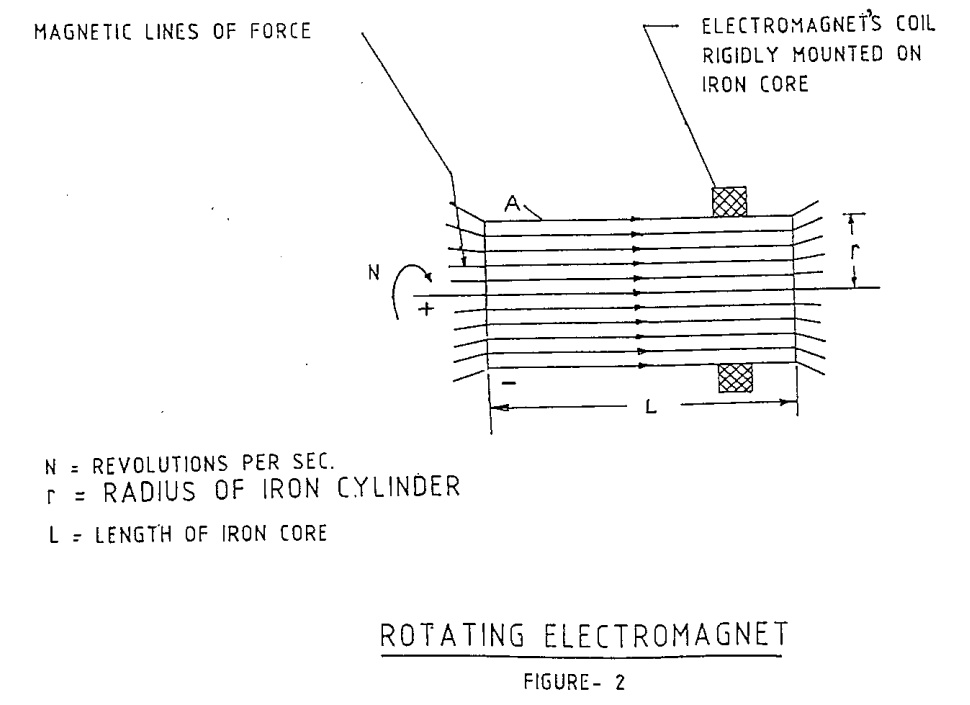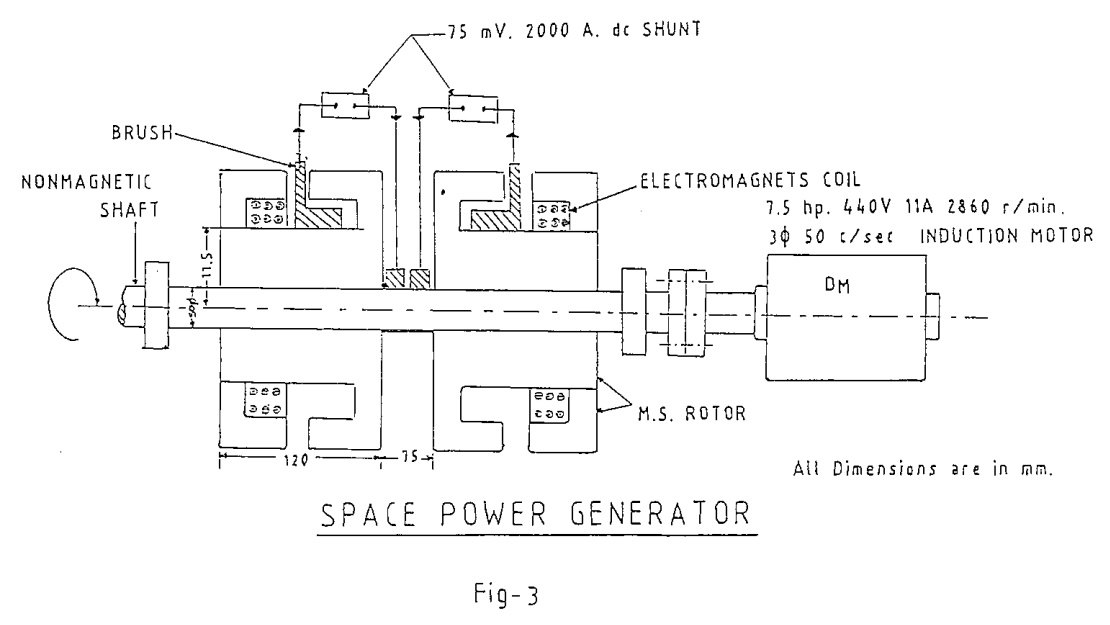## Physics and Technology of the Phenomenon ofSpace Power Generation

Paramahamsa Tewari

### Introduction

Scientific debates on the nature of space (absolute vacuum) around the start of this century took a general view that space serves to transmit fields (electromagnetic, gravitational) and, beyond that, it has no independent existence of its own as an entity that can generate energy in some form which enables it to be termed as a “real” physical entity. In other words, the generally accepted position then taken was that space is an empty extension of “nothingness” without any physical attributes in which fields are somehow transmitted at enormous speed. Many refutations to the above view both experimental and theoretical, and claims on the existence of ether, that is, space with physical attributes, could not provide an alternate theory so as to change the widely accepted position of nonphysical nature of space.

A search for the most basic and universal source of fields of matter in general, and electron in particular, has led to the formulation (by the writer) of space vortex theory (SVT) in which physical space has been postulated,1 and it has been shown that the primary function of space is to generate cosmic matter. Thus as per SVT, quite contrary to the modern view of physics, matter apart from space has no independent existence.### Basics of Space Vortex Theory (SVT)

The absolute vacuum in SVT is a non-material and mobile entity — an incompressible, non-viscous, massless and continuous medium and its dynamical field equations or laws are formulated introducing concept of velocity fields, that is, velocity of space points in a vortex of mobile space. An electron is postulated to be an irrotational vortex of space with a spherical central void of sub-microscopic radius of about ten raised to the power minus eleven centimeter, within which the space with physical attributes develops discontinuity of energy field (Fig. 1). The property of electric charge of electron and its electrostatic field can be shown to be the effect of rotation of space around the electron’s centre. The mass property of electron is seen to be arising due to the creation of a fieldless spherical void (hole) at electron’s centre where space rotates at the limiting speed of light. New equations that quantify mass and charge of electrons and enable the computation of energy for its creation and annihilation are derived. It also follows from the theory that the electron is the only fundamental particle that can build all the other stable particles of matter of the universe.

The generation of mass of electron from the mass-less medium of space is the unique feature of SVT as it does away with the normal trend of scientific convention that every conceivable entity must necessarily have mass if it has to be real, that is, if it has to possess energy. An irrotational vortex of space with maximum speed of rotation less than speed of light will possess zero mass, and yet it will have electric charge energy due to velocity fields produced by space rotation. The creation of mass is essentially creation of void at electron’s centre due to limiting speed of rotation and breakdown of space. The velocity field at a point in the neighbourhood of electron determines the electric potential there. the breakdown of space at electron’s centre gravitationally energizes the whole universe creating gravity field and gravity potential. The decrease in gravity potential at a space point due to oscillation/movement of electron/matter creates electromagnetic (light) effect at that point. When an electron is annihilated, it is the decaying gravity potential at each point in space that is seen as light.

The property of zero viscosity and mobility of space up to speed of light in combination with the central void of electron, results in inertia property of electron in its motion. The inertia property for all matter also arises due to the above effect since the electrons are the fundamental particles of all stable particles of matter.### Fundamental Equations on Electron’s Charge and Mass

Following fundamental equations derived from the void-vortex structure of the electron 1 are relevant to the computation of rotational charge energy produced due to rotation of space in the interatomic region of a rotating electromagnet as shown in Fig. 2.

Refer Fig. 1 which shows spin of space at void-space interface. At the elemental surface, tangential velocity of space is re sin , which increases to its limiting value c at the diametrical section of the interface. The basic definitions for electron’s charge, rest-mass and dielectric constant for vacuum are:

qe = (π/4) (4πre2c)
(1)

where

qe is the electron’s charge
re is the radium of spherical void
c is the light speed in vacuum

Hence, it follows that the dimensions of qe are:

[qe] = L3/T



me = (4π/3)re3c
(2)

where

me is the electron’s rest-mass

Hence, it follows that the dimensions for mass me are:

[me] = L4/T

Dielectric constant for vacuum ϵ0 is given by,

ϵ0 = π/2c
(3)

Dimensions for ϵ0 are:

0] = T/L

Substituting in (1) experimentally determined value, qe = 4.8 x 10-10 CGSE units, and supposing the value of void radius re = 10-11cm.

cm3/s = (16) CGSE
(4)

### Generation of Space Power

As shown in Fig.1, for computation of electron’s charge at the interface, the product of speed of spinning space at the elemental surface and its area dA is taken. This indicates that for all values of VF varying from zero to c, charge is produced. Therefore rotation of cylindrical surface A of an iron core (Fig.2) of an electromagnet will create in its interatomic space rotational charge (qr) given by similar relationship as for electronic charge. Neglecting the area occupied by atomic nuclei and orbital electrons at surface A,

qr = space surface x rotational speed

= (2πrL) (2πrN)

= 4π2r2LN
(5)

Converting qr to CGSE units, from (4) and (5),

qr = 64π2r2LN

Nos. of electrons (Ne) equivalent to qr will be:

Ne = (64π2r2LN/qe)
(6)

Energy in the electrostatic field of Ne electrons is computed as below:

Electrostatic energy (U) of a point-charge as per conventional physics is given by:

U = [qe2/2(4πϵ0)] [1r]0
(7)

Where r, the radial distance from the charge centre, varies from zero to infinity. With void-centre of electron, the minimum value of r is taken as re (and not zero) since void is fieldless zone. (The present difficulty in physics of infinite quantity of energy in the field of a point-charge is avoided with void-centre structure of electron).

Substituting the value of ϵ0 from (3) in (7) and from (1) expressing qe in terms of re and c,

U = (π/4)2 (4πre2c)2 / 2(4π2/2c)re
= (3π/16) (4πre3c/3)c2

Which from (2) becomes:

U = (3π/16)mec2
(8)

Net energy produced from rotational charge from (6) and (8),

E = (3π3/4qe) (mec2) r2LN
(9)

Substituting the values, qe = 4.8 x 10-10 CGSE units, me = 9 x 10-28 gram, c = 3 x 1010 cm/sec in (9),

E = (1.98)πr2LN 10-5 KW sec.
(10)

The electric charge energy developed in any rotating body due to space rotation within the body is given to (10). If the body in rotation is an electrical conductor (iron, copper) then the orbital electrons of the atoms are liberated and will move under the action of a magnetic field to form electrical polarities.### Experimental Proof

The above structure of the electron is substantiated through some recent experiments on electromagnetic induction. These experiments have brought to light a new phenomenon in which electromotive force is generated in an electrical conductor even if there is no relative motion between the conductor and the magnetic field which is unlike the principle behind the operation of modern electrical generators for which relative motion between the conductor and the magnetic field is a must. Further, the electrical power generation in the newly discovered phenomenon does not fully reflect in a proportionate increase of the input power, thereby giving an efficiency of more than unity, and revealing a new vital fact that absolute vacuum in the interatomic space of matter can generate electrical charge and power when it is set in a dynamic state. An experiment somewhat similar to above was done by Michael Faraday in the year 1830. He discovered the above phenomenon as regards the production of electromotive force even when the relative motion between the magnetic field and the conductor was zero. He, however, does not appear to have made measurements on the incremental power ratio from such a device. The writer first learnt of this effect from Bruce De Palma’s letters to him (1978-79),  who made an exhaustive study of this effect and named it as N-effect. The experimental machines he built, he termed as N-Generators.

A series of tests on this unique phenomenon of space power generation have been carried out as outlined briefly in references [2, 3, 4]. The space power generator (SPG) shown in Fig.3 has produced efficiency of electrical power generation to more than 250%, and precise test results on other models show that higher efficiencies for output currents in kilo-amperes are possible.

### Conclusion

Building of space power generators of about 100 KW size should be possible to be achieved with conventional technological methods in the near future.

In addition to the discovery, of a novel technology for power generation, the following crucial change in the scientific and philosophical outlook that would be brought about with the new phenomenon of space power generation are:

(1) The fundamental state of universal energy is eternally latent in the dynamic space of the universe.

(2) The cosmic matter is generated from the energy of space in repeated cycles of creation and annihilation.

(3) Space is not an empty extension but rather the most fundamental entity which alone gives reality to the cosmic world.

The present industrial culture demands a continually increasing growth of electrical power. Since the limited resources of thermal, hydroelectrical and nuclear power cannot last for centuries, the need that has now become vital is to discover a power source which is independent of all material resources, so that a regulated generation of power from the presently known technologies is supplemented to a good extent from this eternal source.

A technological breakthrough, which will enable generation of energy directly from space, is in the offing. Nature has, however, already achieved this conversion from space energy to matter in the generation of the cosmic matter of the universe.

### References

1. Tewari, Paramahamsa. Beyond Matter. Aligarh, India: Print Well Publications, 1984.
2. Tewari, Paramahamsa. "Generation of Electrical Power from Absolute Vacuum by High Speed Rotation of Conducting Magnetic Cylinder." Magnets in Your Future 1:8 (August 1986).
3. Tewari, Paramahamsa. "Interaction of Electrons and Magnetic Field in Space Power Generation Phenomenon." Magnets in Your Future 2:12 (December 1987).
4. Tewari, Paramahamsa. "Violation of Law of Conservation of Charge in Space Power Generation Phenomenon." Jour. Borderland Research 45:5 (Sept-October 1989). <Index>
5. Bruce De Palma, 1187, Coast Village Road, #1-163, Santa Barbara, California 93108, USA.